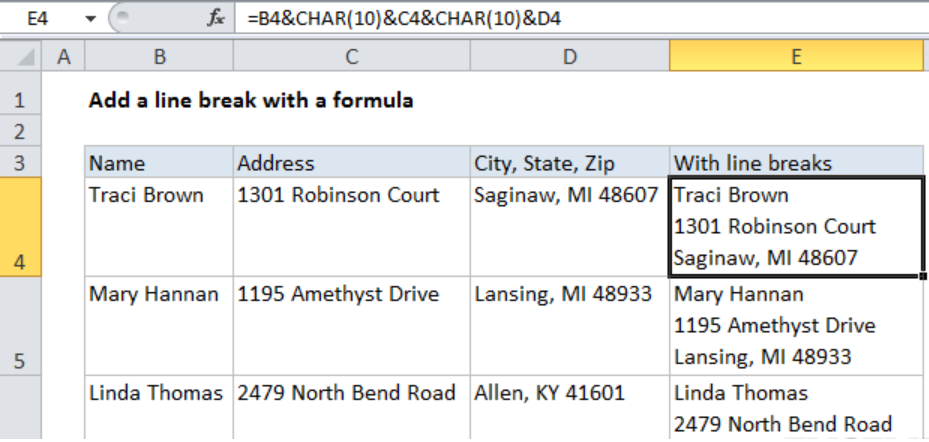Get instant live expert help with Excel or Google Sheets“My Excelchat expert helped me in less than 20 minutes, saving me what would have been 5 hours of work!”

#### Post your problem and you’ll get Expert help in seconds.

Your message must be at least 40 characters
Our professional Expert are available now. Your privacy is guaranteed.

# How to Add a Line Break with Formula in Excel

We can easily add a line break with a formula using the concatenation operator (&) along with the CHAR function in excel. This post will provide a step-by-step guide on how to use the function together with the operator to add a line break with formula in excel.Figure 1: How to add a line break with a formula

## General syntax of the formula

`= “text” &CHAR(10) & “text)`

## Understanding the formula

• To fully understand how this formula works, it is important that we focus on the example in the above figure.
• The formula pieces together the text in cells B4, C4 and D4 using the concatenation operator (&).
• Between each piece, we have the CHAR function which appears with an argument 13. Note that the character code for line break will vary based on the platform. Check the operating system you are using to know the character code to use.
• For this formula to work perfectly, we need to have the text wrap enabled for all the cells that contain line breaks.

## Instant Connection to an Expert through our Excelchat Service

Most of the time, the problem you will need to solve will be more complex than a simple application of a formula or function. If you want to save hours of research and frustration, try our live Excelchat service! Our Excel Experts are available 24/7 to answer any Excel question you may have. We guarantee a connection within 30 seconds and a customized solution within 20 minutes.

### Did this post not answer your question? Get a solution from connecting with the expert.Another blog reader asked this question today on Excelchat:
Solution examplesI have 4 column. when i fill the first 3 column. in 4th column i need a unique id number and i generate unique id number using RANDBETWEEN formula [ =CHAR(RANDBETWEEN(65,90))&CHAR(RANDBETWEEN(65,90))&RANDBETWEEN(1000,9900) ] but i want ..... when i'm filling the 3 column after that 4th column run this formula and generate unique id automatically
Solved by X. Q. in 20 minswhen i fill column A and B then C column generate Unique ID using RandBetween Formula {=CHAR(RANDBETWEEN(65,90))&CHAR(RANDBETWEEN(65,90))&RANDBETWEEN(1000,9900)} ...
Solved by S. Q. in 20 mins## Subscribe to Excelchat.coAnother blog reader asked this question today on Excelchat: